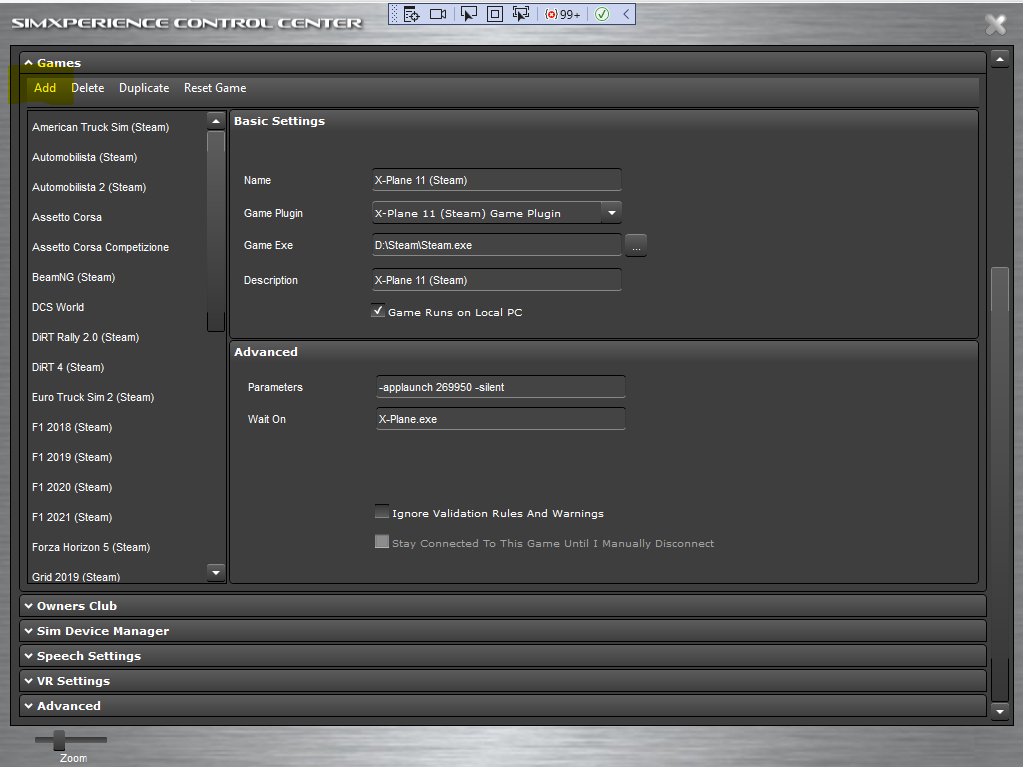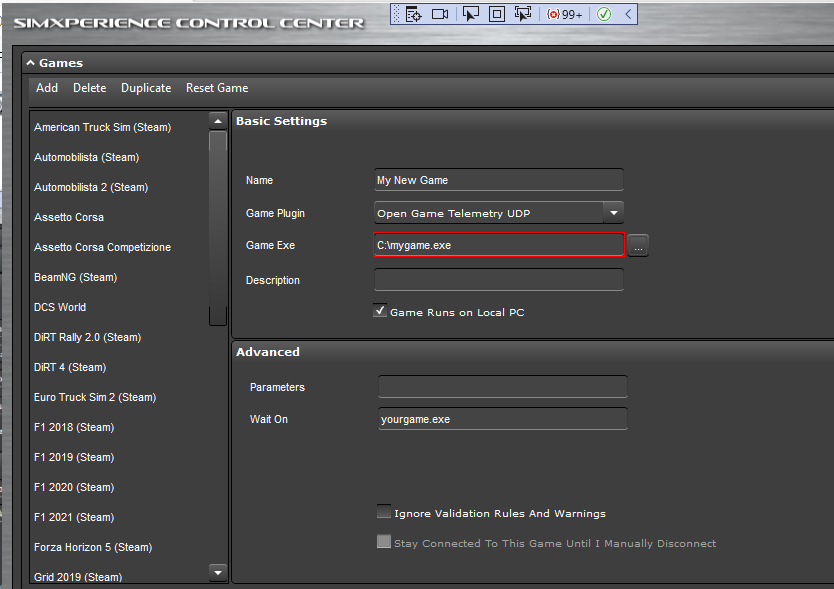## Sim Commander Software Documentation

Last update: 03/31/2022

# Overview

Sim Commander 4 provides several options for 3rd party developers to add Sim Commander support for a currently unsupported game. This tutorial will describe the UDP method.

In this tutorial we'll discuss how to build a helper app that takes telemetry from a currently unsupported game and outputs it in a format understood by Sim Commander. Then we'll setup a Sim Commander game record and profile to consume this UDP telemetry data.

If you are aware of a game that outputs telemetry data either via a plugin or shared memory or UDP, you can contact SimXperience to request official support for the game and avoid this helper app process.

# Writing The Helper App

#### Overview

The purpose of your helper app is to acquire the necessary game telemetry data and output it in the UDP structure and with the units defined below. You can write this app in any language so long as it runs on the Windows 10/11 platform.

#### UDP Data Structure

Sim Commander expects to receive 240 bytes from your helper app on UDP port 10001. Each UDP field is a 4-byte float and ordered as described below.

All items are suggested, but items marked in bold are required for proper functionality.

<float scale="1.0" channel="total_time" />

<float scale="1.0" channel="paused" />

<float scale="1.0" channel="yaw" />

<float scale="1.0" channel="pitch" />

<float scale="1.0" channel="roll" />

<float scale="1.0" channel="yaw_velocity" />

<float scale="1.0" channel="pitch_velocity" />

<float scale="1.0" channel="roll_velocity" />

<float scale="1.0" channel="yaw_acceleration" />

<float scale="1.0" channel="pitch_acceleration" />

<float scale="1.0" channel="roll_acceleration" />

<float scale="1.0" channel="position_x" />

<float scale="1.0" channel="position_y" />

<float scale="1.0" channel="position_z" />

<float scale="1.0" channel="local_velocity_x" />

<float scale="1.0" channel="local_velocity_y" />

<float scale="1.0" channel="local_velocity_z" />

<float scale="1.0" channel="gforce_lateral" />

<float scale="1.0" channel="gforce_longitudinal" />

<float scale="1.0" channel="gforce_vertical" />

<float scale="1.0" channel="speed" />

<float scale="1000.0" channel="suspension_position_bl" />

<float scale="1000.0" channel="suspension_position_br" />

<float scale="1000.0" channel="suspension_position_fl" />

<float scale="1000.0" channel="suspension_position_fr" />

<float scale="1000.0" channel="suspension_velocity_bl" />

<float scale="1000.0" channel="suspension_velocity_br" />

<float scale="1000.0" channel="suspension_velocity_fl" />

<float scale="1000.0" channel="suspension_velocity_fr" />

<float scale="1000.0" channel="suspension_acceleration_bl" />

<float scale="1000.0" channel="suspension_acceleration_br" />

<float scale="1000.0" channel="suspension_acceleration_fl" />

<float scale="1000.0" channel="suspension_acceleration_fr" />

<float scale="1.0" channel="wheel_patch_speed_bl" />

<float scale="1.0" channel="wheel_patch_speed_br" />

<float scale="1.0" channel="wheel_patch_speed_fl" />

<float scale="1.0" channel="wheel_patch_speed_fr" />

<float scale="1.0" channel="throttle_input" />

<float scale="1.0" channel="steering_input" />

<float scale="1.0" channel="brake_input" />

<float scale="1.0" channel="clutch_input" />

<float scale="1.0" channel="gear" />

<float scale="1.0" channel="max_gears" />

<float scale="1.0" channel="engine_rate" />

<float scale="1.0" channel="race_position" />

<float scale="1.0" channel="race_sector" />

<float scale="1.0" channel="sector_time_1" />

<float scale="1.0" channel="sector_time_2" />

<float scale="1.0" channel="brake_temp_bl" />

<float scale="1.0" channel="brake_temp_br" />

<float scale="1.0" channel="brake_temp_fl" />

<float scale="1.0" channel="brake_temp_fr" />

<float scale="1.0" channel="tyre_pressure_bl" />

<float scale="1.0" channel="tyre_pressure_br" />

<float scale="1.0" channel="tyre_pressure_fl" />

<float scale="1.0" channel="tyre_pressure_fr" />

<float scale="1.0" channel="lap" />

<float scale="1.0" channel="laps_completed" />

<float scale="1.0" channel="total_laps" />

<float scale="1.0" channel="lap_time" />

<float scale="1.0" channel="lap_distance" />

<float scale="1.0" channel="track_length" />

<float scale="1.0" channel="last_lap_time" />

<float scale="1.0" channel="max_rpm" />

<float scale="1.0" channel="idle_rpm" />

#### Timing

Sim Commander can accept any data rate from 60Hz - 400Hz for the incoming data stream. Please note that timing consistency is more important than overall speed of the update rate. That is to say that a data stream that arrives at 60Hz with no timing jitter is better than a jittery 400Hz UDP stream for our purposes.

#### Units

• Total_time is the time since the session began in seconds. Used for derivative calculations. Must be accurate.

• Yaw, Pitch and Roll are expressed in radians

• Yaw, Pitch and Roll Velocity are expressed in radians/second

• Yaw, Pitch and Roll Acceleration are expressed in radians/second squared

• G-Force values are expressed in G

• Speed is the vehicles linear velocity in m/s

• Suspension positions are expressed in meters

• Suspension velocities are expressed in m/s

• Suspension accelerations are expressed in m/s squared

• Gear is the current gear number. 0 for neutral. -1 for reverse.

• Engine rate is expressed in radians/second.

• Lap time is the current lap time expressed in seconds

• Lap distance is the distance of the track in meters.

• Local Velocity X/Y/Z is the linear velocity of the car in car local space (in meters)

• Total distance is the race distance in meters.

# Adding a Game Record

#### Introduction

Each Sim Commander profile has an associated game record. The game record tells Sim Commander what exe to launch and what exe to look for to know that the game is running. A game record also tells Sim Commander which plugin to use to interpret inbound telemetry data. You must create a game record for your currently unsupported game following the steps below.

#### Adding the Game Record

1. Click the Settings (gear) icon from the main screen

2. Expand the game section.

3. Click 'Add' to add a new game record4. Give your game record a name, then specify the 'Open Game Telemetry - UDP' plugin, the Game Exe to lanch and the exe name to wait on to determine the game is running.In this example, mkygame.exe is launched by Sim Commander and Sim Commander will wait until it finds yourgame.exe running to initialize the device profiles. In many cases, the Game exe and Wait On may be the same.

In a later step, we will assign this game record to a profile.

# Creating a Profile

#### Introduction

A Sim Commander profile contains the effects that will be output to each device as well as a link to a game record so Sim Commander knows how to launch the game and how to interpret the incoming data.

#### Create the Profile

The simplest way to create a profile is to duplicate a profile from a similar game. For example, if you are working with a new racing game, you can duplicate the Dirt 4 (or other) profile by hovering over the profile and clicking the 'Duplicate' button.